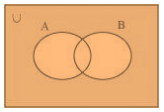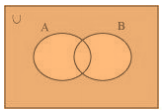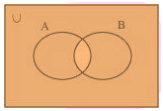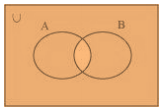• +91 9971497814
• info@interviewmaterial.com

# Chapter 1 Sets Ex-1.5 Interview Questions Answers

### Related Subjects

Question 1 :
Let U = {1, 2, 3; 4, 5, 6, 7, 8, 9}, A = {1, 2, 3, 4}, B = {2, 4, 6, 8} and C = {3, 4, 5, 6}. Find
(i) A’
(ii) B’
(iii) (A U C)’
(iv) (A U B)’
(v) (A’)’
(vi) (B – C)’

It is given that
U = {1, 2, 3, 4, 5, 6, 7, 8, 9}
A = {1, 2, 3, 4}
B = {2, 4, 6, 8}
C = {3, 4, 5, 6}
(i) A’ = {5, 6, 7, 8, 9}
(ii) B’ = {1, 3, 5, 7, 9}
(iii) A U C = {1, 2, 3, 4, 5, 6}
So we get
(A U C)’ = {7, 8, 9}
(iv) A U B = {1, 2, 3, 4, 6, 8}
So we get
(A U B)’ = {5, 7, 9}
(v) (A’)’ = A = {1, 2, 3, 4}
(vi) B – C = {2, 8}
So we get
(B – C)’ = {1, 3, 4, 5, 6, 7, 9}

Question 2 :
If U = {a, b, c, d, e, f, g, h}, find the complements of the following sets:
(i) A = {a, b, c}
(ii) B = {d, e, f, g}
(iii) C = {a, c, e, g}
(iv) D = {f, g, h, a}

(i) A = {a, b, c}
So we get
A’ = {d, e, f, g, h}
(ii) B = {d, e, f, g}
So we get
B’ = {a, b, c, h}
(iii) C = {a, c, e, g}
So we get
C’ = {b, d, f, h}
(iv) D = {f, g, h, a}
So we get
D’ = {b, c, d, e}

Question 3 :
Taking the set of natural numbers as the universal set, write down the complements of the following sets:
(i) {x: x is an even natural number}
(ii) {x: x is an odd natural number}
(iii) {x: x is a positive multiple of 3}
(iv) {x: x is a prime number}
(v) {x: x is a natural number divisible by 3 and 5}
(vi) {x: x is a perfect square}
(vii) {x: x is perfect cube}
(viii) {x: x + 5 = 8}
(ix) {x: 2x + 5 = 9}
(x) {x: x ≥ 7}
(xi) {x: x ∈ N and 2x + 1 > 10}

We know that
U = N: Set of natural numbers
(i) {x: x is an even natural number}´ = {x: x is an odd natural number}
(ii) {x: x is an odd natural number}´ = {x: x is an even natural number}
(iii) {x: x is a positive multiple of 3}´ = {x: x ∈ N and x is not a multiple of 3}
(iv) {x: x is a prime number}´ ={x: x is a positive composite number and x = 1}
(v) {x: x is a natural number divisible by 3 and 5}´ = {x: x is a natural number that is not divisible by 3 or 5}
(vi) {x: x is a perfect square}´ = {x: x ∈ N and x is not a perfect square}
(vii) {x: x is a perfect cube}´ = {x: x ∈ N and x is not a perfect cube}
(viii) {x: x + 5 = 8}´ = {x: x ∈ N and x ≠ 3}
(ix) {x: 2x + 5 = 9}´ = {x: x ∈ N and x ≠ 2}
(x) {x: x ≥ 7}´ = {x: x ∈ N and x < 7}
(xi) {x: x ∈ N and 2x + 1 > 10}´ = {x: x ∈ N and x ≤ 9/2}

Question 4 :
If U = {1, 2, 3, 4, 5,6,7,8, 9}, A = {2, 4, 6, 8} and B = {2, 3, 5, 7}. Verify that
(i) (A U B)’ = A’ ∩ B’
(ii) (A ∩ B)’ = A’ U B’

It is given that
U = {1, 2, 3, 4, 5,6,7,8, 9}
A = {2, 4, 6, 8}
B = {2, 3, 5, 7}
(i) (A U B)’ = {2, 3, 4, 5, 6, 7, 8}’ = {1, 9}
A’ ∩ B’ = {1, 3, 5, 7, 9} ∩ {1, 4, 6, 8, 9} = {1, 9}
Therefore, (A U B)’ = A’ ∩ B’.
(ii) (A ∩ B)’ = {2}’ = {1, 3, 4, 5, 6, 7, 8, 9}
A’ U B’ = {1, 3, 5, 7, 9} U {1, 4, 6, 8, 9} = {1, 3, 4, 5, 6, 7, 8, 9}
Therefore, (A ∩ B)’ = A’ U B’.

Question 5 :
Draw appropriate Venn diagram for each of the following:
(i) (A U B)’
(ii) A’ ∩ B’
(iii) (A ∩ B)’
(iv) A’ U B’

(i) (A U B)’(ii) A’ ∩ B’(iii) (A ∩ B)’(iv) A’ U B’Question 6 : Let U be the set of all triangles in a plane. If A is the set of all triangles with at least one angle different from 60°, what is A’?

Answer 6 : A’ is the set of all equilateral triangles.

Question 7 :
Fill in the blanks to make each of the following a true statement:
(i) A U A’ = ……..
(ii) Φ′ ∩ A = …….
(iii) A ∩ A’ = …….
(iv) U’ ∩ A = …….

(i) A U A’ = U
(ii) Φ′ ∩ A = U ∩ A = A
So we get
Φ′ ∩ A = A
(iii) A ∩ A’ = Φ
(iv) U’ ∩ A = Φ ∩ A = Φ
So we get
U’ ∩ A = Φ

Todays Deals### Chapter 1 Sets Ex-1.5 Contributorskrishan

Name:
Email:

# Latest News# 9000 interview questions in different categories